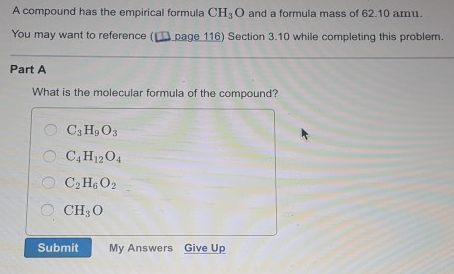# Problem: A compound has the empirical formula CH3O and a formula mass of 62.10 amu. What is the molecular formula of the compound? (a) C3H9O3 (b) C4H12O4 (c) C2H6O2 (d) CH3O

###### FREE Expert Solution

CH3O has a molecular mass of 31.01 g/mol

97% (348 ratings)###### Problem Details

A compound has the empirical formula CH3O and a formula mass of 62.10 amu. What is the molecular formula of the compound?

(a) C3H9O3

(b) C4H12O4

(c) C2H6O2

(d) CH3OFrequently Asked Questions

What scientific concept do you need to know in order to solve this problem?

Our tutors have indicated that to solve this problem you will need to apply the Molecular Formula concept. You can view video lessons to learn Molecular Formula. Or if you need more Molecular Formula practice, you can also practice Molecular Formula practice problems.

What professor is this problem relevant for?

Based on our data, we think this problem is relevant for Professor Kretchmer & Shepler's class at GT.